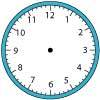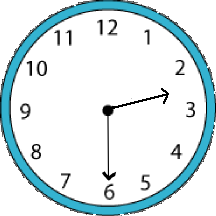You may also likeOn Time

On a clock the three hands - the second, minute and hour hands - are on the same axis. How often in a 24 hour day will the second hand be parallel to either of the two other hands?Right Time

At the time of writing the hour and minute hands of my clock are at right angles. How long will it be before they are at right angles again?Estimating Angles

How good are you at estimating angles?

Half Past Two

Age 11 to 14 Short Challenge Level:In moving from one number on the clock face to the next, a hand moves $360 \div 12 ^\circ = 30 ^\circ$.
At 2.30, the hour hand will be exactly half way between the $2$ and the $3$, and the minute hand will be exactly on the $6$.
So the angle between the two hands will be $3 \times 30^\circ + 15^\circ = 105^\circ$.
This problem is taken from the UKMT Mathematical Challenges.
You can find more short problems, arranged by curriculum topic, in our short problems collection.